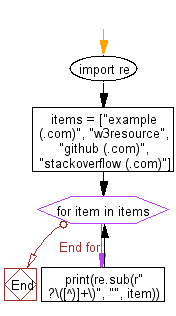﻿ Python: Remove the parenthesis area in a string - w3resource# Python: Remove the parenthesis area in a string

## Python Regular Expression: Exercise-50 with Solution

Write a Python program to remove the parenthesis area in a string.

Sample Solution:-

Python Code:

``````import re
items = ["example (.com)", "w3resource", "github (.com)", "stackoverflow (.com)"]
for item in items:
print(re.sub(r" ?\([^)]+\)", "", item))
```
```

Sample Output:

```example
w3resource
github
stackoverflow
```

Pictorial Presentation:Flowchart:## Visualize Python code execution:

The following tool visualize what the computer is doing step-by-step as it executes the said program:

Python Code Editor:

Have another way to solve this solution? Contribute your code (and comments) through Disqus.

What is the difficulty level of this exercise?

Test your Python skills with w3resource's quiz

﻿

## Python: Tips of the Day

For-else construct useful when searched for something and find it:

```# For example assume that I need to search through a list and process each item until a flag item is found and
# then stop processing. If the flag item is missing then an exception needs to be raised.

for i in mylist:
if i == theflag:
break
process(i)
else:
raise ValueError("List argument missing terminal flag.")
```